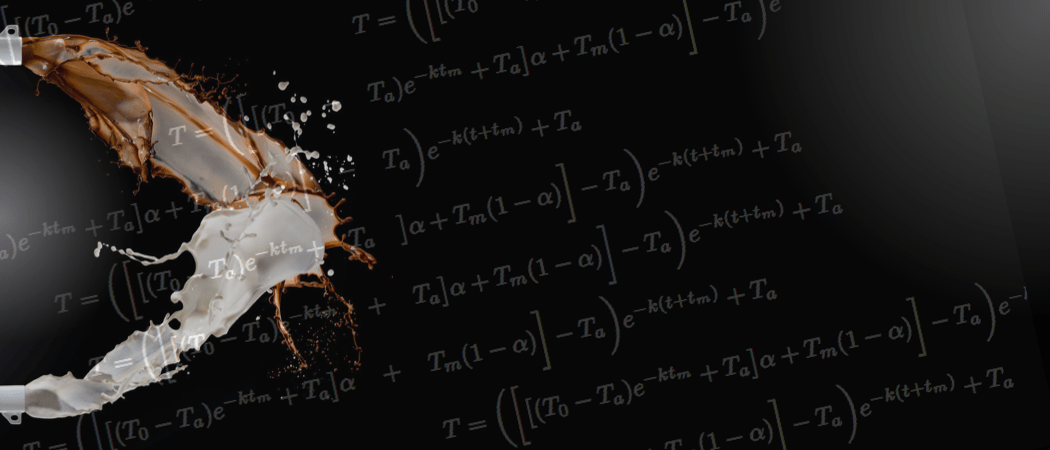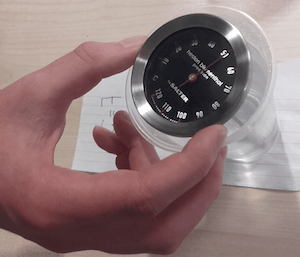# The mathematics of tea-making

When-to-add-milk confusion and other related mattersDue to the immense popularity of tea, many heated arguments have been started in kitchens, at dinner tables and indeed on the internet concerning the proper way of making it. There is a lot of confusion surrounding tea–making, including—but not limited to—the question of the right brewing temperature, the amount of milk one should add and the correct order of adding milk and hot water. Clearly there’s way too many variables and one can easily get lost in all that madness. On top of this, looking for advice on the internet results in an inundation of contradictory advice and very hysterical arguments. So let’s put all the emotions aside and seek refuge in the realms of maths and science.Recently, students from the University of Leicester came up with a formula for the perfect brew. According to them, one should add 200ml of boiled water, let it brew for 2 minutes, then add 10ml of milk and wait 6 minutes for it to reach its optimum temperature of 60°C. Which is all very nice but not everyone can be bothered to measure the exact amount of milk and water and, ultimately, we don’t live in an ideal world where one can start making a cup of tea safe in the knowledge that there will be no interruption halfway through. Since we at Chalkdust are mainly concerned with practical consumer advice, let us consider the scenario described below (in the discussion that follows we shall assume that milk is added after the water. Partly because if you don’t, it would make the whole article meaningless, but mostly because any other practice is simply wrong and should not be encouraged in any way).

### The problem

We’ve all been there, you boil the water, pour it into the cup and suddenly the doorbell rings. It could be the postman, your neighbour asking for some sugar, or a religious enthusiast trying to save you from eternal damnation. Either way, it’s going to be a few minutes before you can enjoy your tea. Obviously, you would like to maximise your chances of your tea still being nice and hot upon your return. What should you do? Add milk now and answer the door or pour the milk later once the nuisance business has been taken care of?

### Building the model

To start answering the question, we need to have an idea of how an object cools down. According to Newton’s law of cooling, the rate of cooling of an object is negatively proportional to the difference between its temperature and the temperature of the environment.

$$\frac{\partial T}{\partial t} = -k(T-T_a),$$

where T is the temperature of the object in question (in our case a cup of tea), $T_a$ is the temperature of the environment and k is a positive constant. Hence given the initial temperature $T_0$ we can easily obtain a solution to the differential equation

$$T = (T_0 – T_a)e^{-kt} + T_a.$$This model assumes that all the thermodynamic properties of the system, (such as the heat capacity, thermal conductivity, etc) can be ‘hidden’ in that one constant k. But what is its value? The best way to find out is to perform some experiments. So we bought some thermometers, put the kettle on and made some measurements. We measured the temperature of the room (which was quite cold, only 16°C!) and made a note of the temperature of the boiled water in 1-minute intervals. Then the next step is relatively simple. We need to fit a value for k that minimises the error between the measured temperatures and the values predicted by the model. For our purposes, we chose the sum squared error as our measure and asked Microsoft Excel do the rest of the work for us. This gave us a value of k equal to roughly 0.03.

https://plot.ly/~r.kohulak/36/observations-vs-model/

So far so good. We still need to address the issue of adding milk. Let’s start by looking at a more general case. Suppose we have two liquids at different temperatures $T_1$ and $T_2$. Now let’s assume that when we pour these into one container they will instantly mix. This is partially justified by the video below where red fluid mixes with blue one almost instantly to produce a purple colour. Hence one can assume that the temperatures will ‘mix’ and that the final temperature will be an average of the temperatures of the two liquids weighted by their relative volumes in the mixture. So the final temperature is given by

$$T = T_1\alpha + T_2(1-\alpha),$$

where $\alpha$ is the volume of liquid one relative to the whole mixture.

Let’s now piece it all together. We denote $T_b$ as the temperature of the tea just before we add milk to it and $T_m$ as the temperature of the milk. Then the temperature after the milk has been added should be

$$T_{\text{after}} = T_b\alpha + T_m(1-\alpha).$$

Supposing that we add milk at time $t_m$ then $T_b$ is given by

$$T_b = (T_0 – T_a)e^{-kt_m} + T_a.$$

Then according to the model, after the milk has been poured in the temperature of the tea follows

$$T = (T_{\text{after}} – T_a)e^{-k(t + t_m)} + T_a.$$

Hence putting all this together we get the final model

$$T = \bigg(\bigg[\big[(T_0 – T_a)e^{-kt_m} + T_a\big]\alpha + T_m(1-\alpha)\bigg] – T_a\bigg)e^{-k(t + t_m)} + T_a.$$

### Experimentation, results and a lot of tea-making

But does this actually work? To find out we once again grabbed the thermometers and boiled some water. We tested three scenarios: $t_m$ = 0, 5 and 10. Due to lack of time and tiredness, we decided to perform all three of them at once. However, due to a lack of manpower and equipment we could not measure all three of them at exactly the same time, so we measured with a 20s time delay between the three cases. After having done that, we randomised the positions of the cups and their associated time delay and repeated the experiments.

The results are plotted in the figure above. It is clear that the model gives a slightly wrong temperature for the smaller times. This is probably due to the fact that the cooling effects of the containers are not accounted for. However, putting that aside, the predictions are NOT TOO BAD. According to the measurements (and indeed the model), putting in the milk earlier resulted in higher final temperatures.

https://plot.ly/~r.kohulak/33/comparison-of-measurements-against-model-prediction-with-milk/

So while the maths needed lots of constants and parameter values, our advice to you—unlike that of the students at the University of Leicester—is simple: the next time the doorbell rings while you are making your daily brew, add the milk immediately!Rudolf Kohulák is a PhD student at UCL working on the modelling of freeze-drying processes.
+ More articles by Rudolf